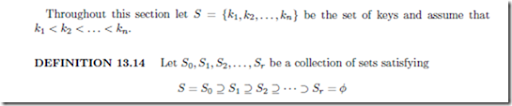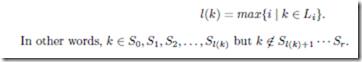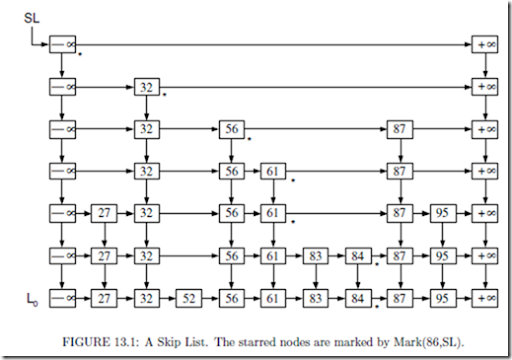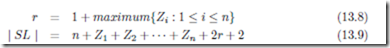# Randomized Dictionary Structures:Skip Lists.

## Skip Lists

Linked list is the simplest of all dynamic data structures implementing a Dictionary. How- ever, the complexity of Search operation is O(n) in a Linked list. Even the Insert and Delete operations require O(n) time if we do not specify the exact position of the item in the list. Skip List is a novel structure, where using randomness, we construct a number of progressively smaller lists and maintain this collection in a clever way to provide a data structure that is competitive to balanced tree structures. The main advantage oﬀered by skip list is that the codes implementing the dictionary operations are very simple and resemble list operations. No complicated structural transformations such as rotations are done and yet the expected time complexity of Dictionary operations on Skip Lists are quite comparable to that of AVL trees or splay trees. Skip Lists are introduced by Pugh .Then, we say that the collection S0, S1, S2,…, Sr deﬁnes a leveling with r levels on S. The keys in Si are said to be in level i, 0 i r. The set S0 is called the base level for the leveling scheme. Notice that there is exactly one empty level, namely Sr . The level number l(k) of a key k S is deﬁned byFor an eﬃcient implementation of the dictionary, instead of working with the current set S of keys, we would rather work with a leveling of S. The items of Si will be put in the increasing order in a linked list denoted by Li. We attach the special keys at the beginning and +at the end of each list Li as sentinels. In practice, is a key value that is smaller than any key we consider in the application and +denotes a key value larger than all the possible keys. A leveling of S is implemented by maintaining all the lists L0, L1, L2,…, Lr with some more additional links as shown in Figure 13.1. Speciﬁcally, the box containing a key k in Li will have a pointer to the box containing k in Li1. We call such pointers descent pointers. The links connecting items of the same list are called horizontal pointers. Let B be a pointer to a box in the skip list. We use the notations Hnext[B], and Dnext[B], for the horizontal and descent pointers of the box pointed by B respectively. The notation key[B] is used to denote the key stored in the box pointed by B.

The name of the skip list is nothing but a pointer to the box containing in the rth level as shown in the ﬁgure. From the Figure 13.1 it is clear that Li has horizontal pointers that skip over several intermediate elements of Li1. That is why this data structure is called the Skip List.How do we arrive at a leveling for a given set? We may construct Si+1 from Si in a systematic, deterministic way. While this may not pose any problem for a static search problem, it will be extremely cumbersome to implement the dynamic dictionary operations. This is where randomness is helpful. To get Si+1 from Si, we do the following. For each element k of Si toss a coin and include k in Si+1 iﬀ we get success in the coin toss. If the coin is p-biased, we expect | Si+1 | to be roughly equal to p | Si |. Starting from S, we may repeatedly obtain sets corresponding to successive levels. Since the coin tosses are independent, there is another useful, and equivalent way to look at our construction. For each key k in S, keep tossing a coin until we get a failure. Let h be the number of successes before the ﬁrst failure. Then, we include k in h further levels treating S = S0 as the base level. In other words, we include k in the sets S1, S2,…, Sh. This suggests us to deﬁne a random variable Zi for each key ki S0 to be the number of times we toss a p-biased coin before we obtain a failure. Since Zi denotes the number of additional copies of ki in the skip list, the value maximum{Zi : 1 i n} deﬁnes the highest nonempty level. Hence, we have,where r is the number of levels and | SL | is the number of boxes or the space complexity measure of the Skip List. In the expression for | SL | we have added n to count the keys in the base level and 2r + 2 counts the sentinel boxes containing +and in each level.